# CREST Olympiads - CMO PDF Sample Papers for Class 7

Class 7 sample paper & practice questions for CREST Mathematics Olympiad (CMO) level 1 are given below. Syllabus for level 1 is also mentioned for these exams. You can refer these sample paper & quiz for preparing for the CMO exam.#### Resources:

##### Sample Questions from Olympiad Success:
 Q.1 Q.2 Q.3 Q.4 Q.5 Q.6 Q.7 Q.8 Q.9 Q.10
 Q.1 Find the order of rotational symmetry in the figure given below.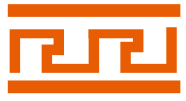a) 4 b) 2 c) 1 d) Infinitely many
 Q.2 The bar graph given below shows the sales of books (in thousand number) from six branches of a publishing company during two consecutive years 2000 and 2001. What is the average sales of all the branches (in thousand numbers) for the year 2000?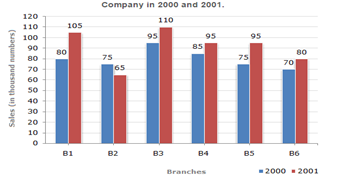a) 73 b) 80 c) 88 d) 83
 Q.3 In fig, AB|| CD, ∠APQ = 50° and ∠PRD = 130°. Find the value of ∠QPR.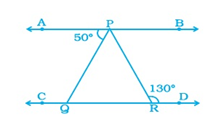a) 130° b) 50° c) 80° d) 30°
 Q.4 Name the solid that has two opposite identical faces and other faces as parallelogram. a) Pyramid b) Prism c) Sphere d) Cone
 Q.5 Which of the following fractions is greater than 3/4 and less than 5/6? a) 1/2 b) 4/5 c) 2/3 d) 9/10
 Q.6 Find the equivalent fraction of 4/7 with numerator 28. a) 28/41 b) 28/49 c) 28/47 d) 28/45
 Q.7 The sum of the ages of a football coach and his two team members is 100. His age will be the sum of the ages of the two team members in 12 years' time. How old is the football coach? a) 56 b) 28 c) 60 d) 30
 Q.8 Choose a figure which would most closely resemble the unfolded form of Figure (Z).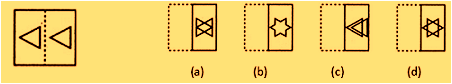a) a b) b c) c d) d
 Q.9 Select the figure which satisfies the same conditions of placement of the dots as in figure.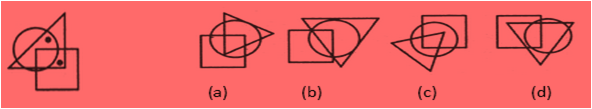a) a b) b c) c d) d
 Q.10 Evaluate. 28% of 450 + 45% of 280. a) 232 b) 262 c) 242 d) 252Sample PDF of CREST Olympiads - CREST Mathematics Olympiad (CMO) PDF Sample Papers for Class 7:

 Q.1 )b Q.2 )b Q.3 )c Q.4 )b Q.5 )b Q.6 )b Q.7 )a Q.8 )d Q.9 )d Q.10 )d

Q.1 : b | Q.2 : b | Q.3 : c | Q.4 : b | Q.5 : b | Q.6 : b | Q.7 : a | Q.8 : d | Q.9 : d | Q.10 : d